## Access NCERT Solutions for Class 6 Maths Chapter 14: Practical Geometry Exercise 14.4

### Access NCERT Solutions for Class 6 Maths Chapter 14: Practical Geometry Exercise 14.4

1. Draw any line segment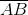. Mark any point M on it. Through M, draw a perpendicular to. (use ruler and compasses)

Solutions:

(1) Draw a line segmentand mark a point M on it.(2) Taking M as centre and a convenient radius, construct an arc intersecting the line segmentat points C and D respectively.(3) By taking centres as C and D and radius greater than CM, construct two arcs such that they intersect each other at point E.(4) Join EM. Now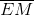is perpendicular to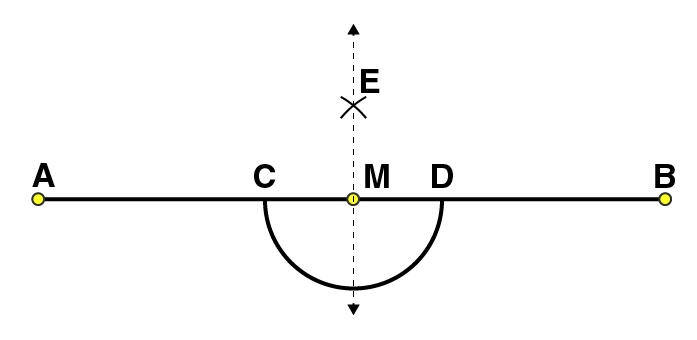2. Draw any line segment. Take any point R not on it. Through R, draw a perpendicular to. (use ruler and set-square)

Solutions:

(1) Draw a given line segment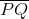and mark a point R outside the line segment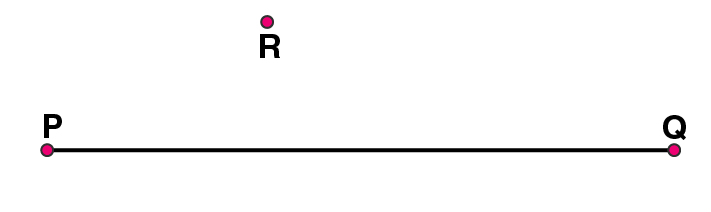(2) Place a set square onsuch that one of its right angles arm aligns along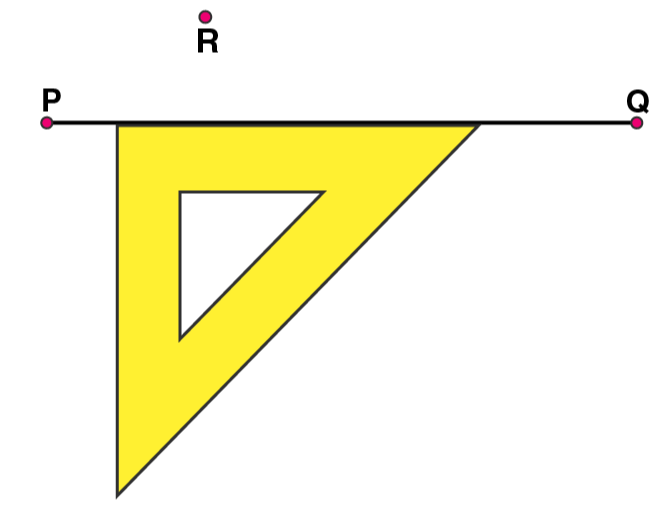(3) Now, place the ruler along the edge opposite to right angle of set square.(4) Hold the ruler fixed. Slide the set square along the ruler such that the point R touches the other arm of set square.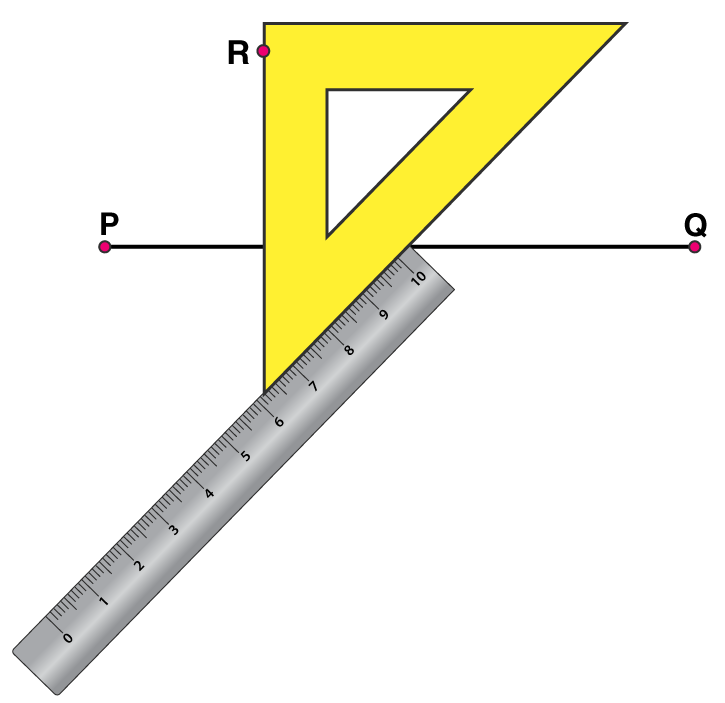(5) Draw a line along this edge of set square which passes through point R. Now, it is the required line perpendicular to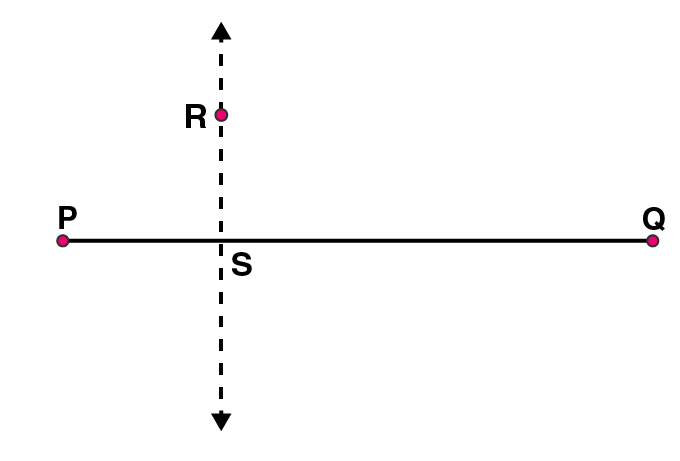3. Draw a line l and a point X on it. Through X, draw a line segment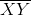perpendicular to l.

Now draw a perpendicular to XY at Y. (use ruler and compasses)

Solutions:

(1) Draw a line l and mark a point X on it.(2) By taking X as centre and with a convenient radius, draw an arc intersecting the line l at points A and B respectively.(3) With A and B as centres and a radius more than AX, construct two arcs such that they intersect each other at point Y.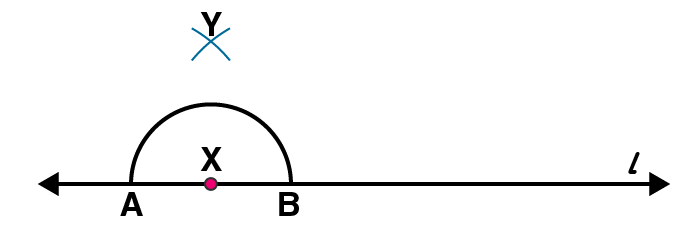(4) Join XY. Hereis perpendicular to lSimilarly, by taking C and D as centres and radius more than CY, construct two arcs intersecting at point Z. Join ZY. The line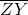is perpendicular toat Y